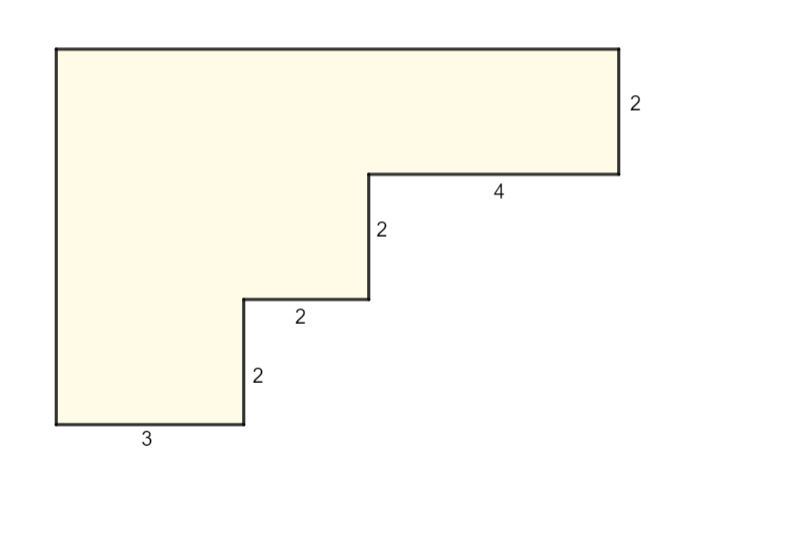Perimeter homework Help at TutorEye

## Top Questions

t schedule 3 people for the day my % scheduled is 78.57% My question is how did the computer come up with the 78.57%?
View More

I would love to get a written solution. I did the other proof tree that you can see in the attaached files. So its supposed to look like that Thank You.
View More

xtbook. i really need in in half an hour
View More

## Perimeter:

The perimeter of a closed shape can be defined as the total length of its outer boundary. Perimeter is the measure of distance we get after summing up the length of the boundary of a shape. The standard unit to measure the perimeter are miles, yards, feet, inches. And, kilometers, meters, and centimeters.

 Perimeter of Quadrilateral Sum of all the sides. Perimeter of Triangle Sum of all the sides. Perimeter of Circle πd=2πr Perimeter of Rectangle 2 (l+w)

## Perimeter Sample Questions:

Question 1: Find the perimeter of a rectangular object having a length of 10 cm, and a width of 4 cm.

Explanation: Use the perimeter of rectangle formula.

Question 2: Find the perimeter of the following shape: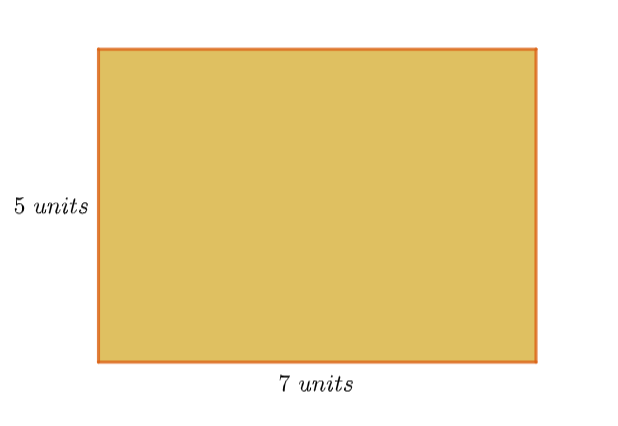Explanation: Use the perimeter of rectangle formula.

Question 3: Calculate the perimeter of the following shape: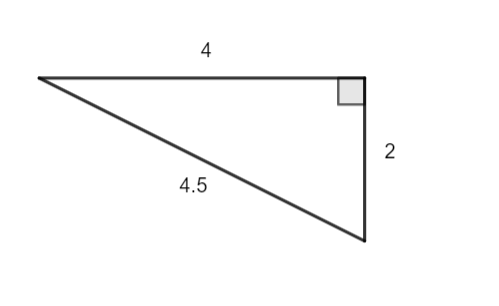Explanation: Use the perimeter of triangle formula.

Question 4: Find the perimeter of the circle having a radius of 3 in.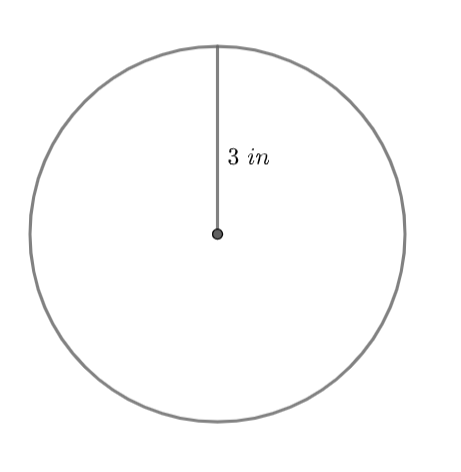Explanation: Use the perimeter of circle formula.

Question 5: The following figure shows the dimension of a triangular plot. Find the total cost of fencing, if available fence costs \$3 per foot.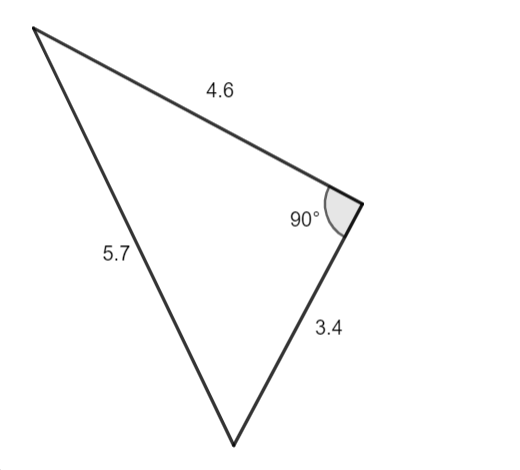Explanation: Use the perimeter of triangle formula.

Question 6: Find the width of a rectangular farm, if the perimeter is 250 yd, and the length is 70 yd.

Explanation: Use the perimeter of rectangle formula.

Question 7: Find the cost of fencing a circular ground having a diameter of 68 m. Given wire fencing costs \$10 per meter. Use π=3.14. Round your answer to the nearest USD.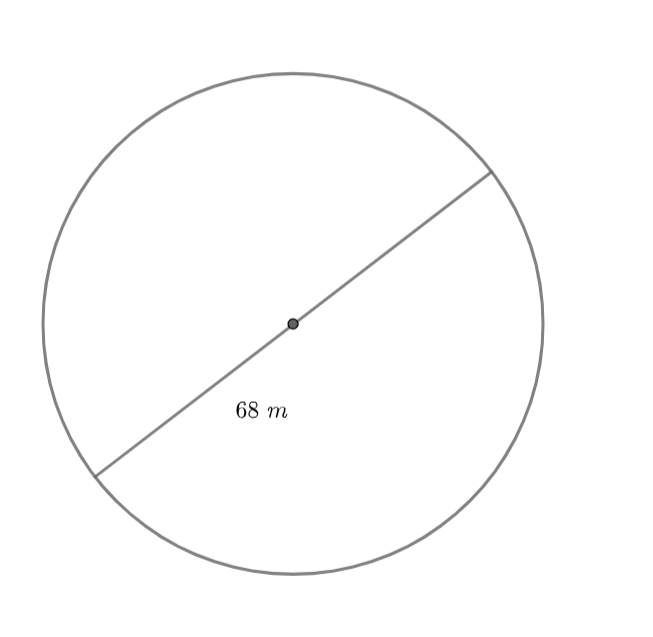Explanation: Use the perimeter of circle formula.

Question 8: Find the length of a rectangular farm, if the perimeter is 315 m, and the width is 52.5 m.

Explanation: Use the perimeter of rectangle formula.

Question 9: Find the perimeter of the following shape: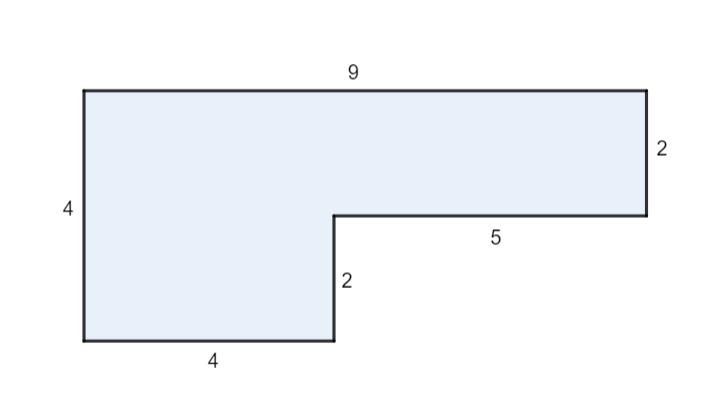Explanation: Use the perimeter of triangle formula.

Question 10: Find the perimeter of the following shape: